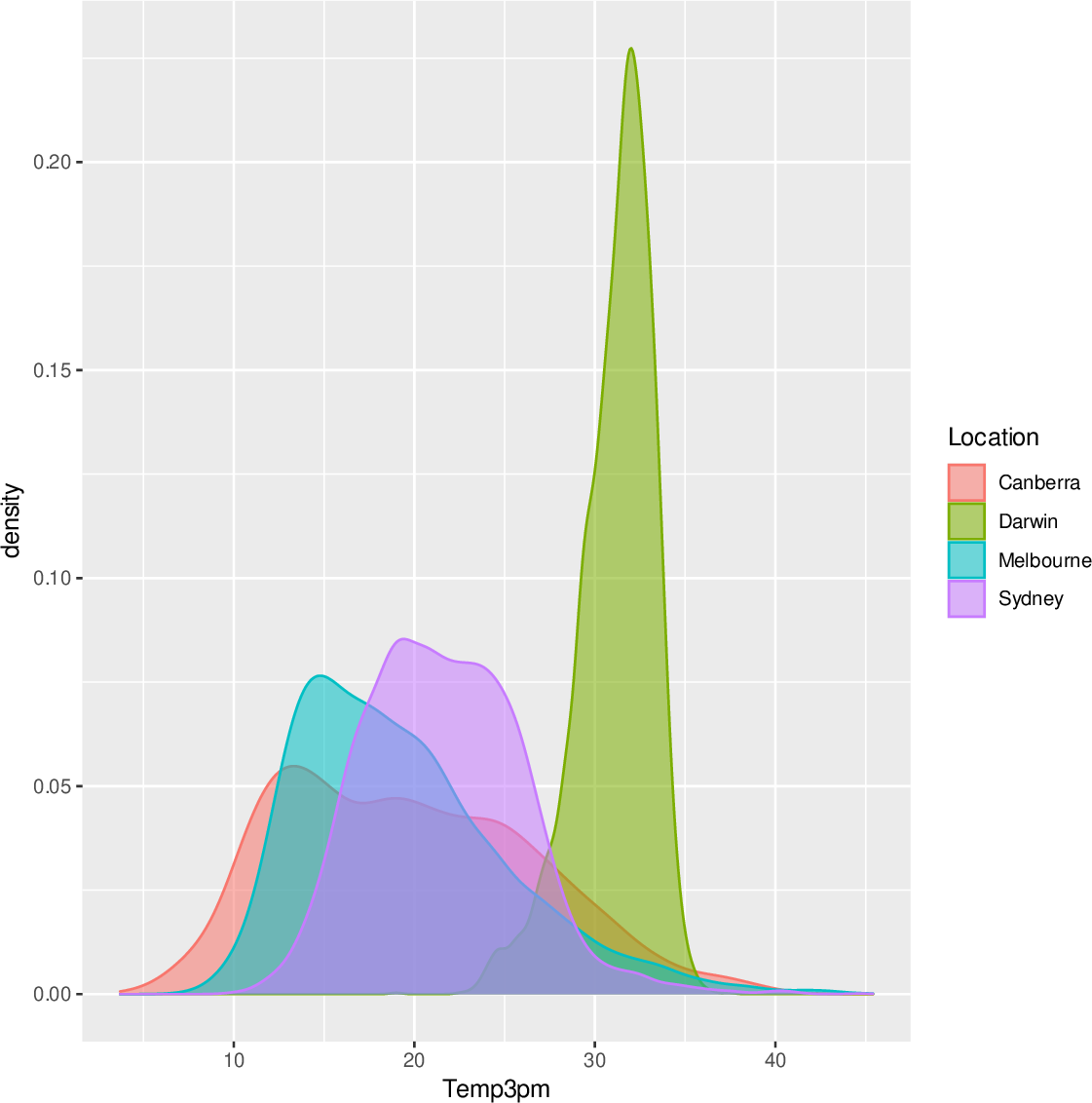Data Science Desktop Survival Guide by Graham WilliamsDesktop Survival Project Home Preface Data Science Introducing R R Constructs R Tasks R Strings R Read, Write, and Create Data Template Data Exploration Data Wrangling Data Visualisation Statistics ML Template ML Scenarios ML Activities ML Applications ML Algorithms Cluster Analysis Decision Trees Computer Vision Graph Data Privacy Literate Data Science Coding with Style Resources Bibliography Index

## Sample Figure

Raw To include figures generated by R in our document we simply add plotting commands to the code chunk. Here for example is R code to generate a simple density plot of the 3pm temperature in 4 cities over a year. We use ggplot2 to generate the figure.

 # Load packages from the local library into the R session. library(rattle)   # For the weatherAUS dataset. library(ggplot2)  # To generate a density plot. # Identify cities of interest. cities <- c("Canberra", "Darwin", "Melbourne", "Sydney") # Generate the plot. weatherAUS %>%   subset(Location %in% cities & ! is.na(Temp3pm)) %>%   ggplot(aes(x=Temp3pm, colour=Location, fill=Location)) +   geom_density(alpha=0.55) In the source document (the .Rnw file) the above R code is actually inserted between the chunk begin and end marks within the document itself. Those marks are:
 ```<>= ... R code ... @ ```

Notice the use of eval=FALSE, which allows the R code to be included in the text of the final document, as it is above, but will not yet generate the plot to be included in the figure. We leave that for a little later.

The code chunk begins by attaching the requisite packages: rattle to access the weatherAUS dataset; and ggplot2 for the function to generate the actual plot.

The four cities we wish to plot are then identified, and we generate a modeltools::subset() of the weatherAUS dataset containing just those cities. We pass the subset on to ggplot2::ggplot() and identify Temp3pm for the x-axis, using location to colour and fill the plot. We add a layer to the figure containing a density plot with a level of transparency specified as an alpha= value. We can see the figure below.We include the figure in the final document as above simply by removing the eval=FALSE from the previous code chunk. Thus the R code is evaluated and a plot is generated. We have actually replaced the eval=FALSE with echo=FALSE so as not to print the R code a second time.

We do not actually need to rewrite the R code again in a second chunk, given the code has already been provided in the first chunk on the previous page. We use a feature of knitr where an empty chunk having the same name as a previous chunk is actually a reference to that previous chunk. Thus in our source .Rnw text document we add the following two lines. This is effectively replaced by the R code from the previous block of the same name.

 ```<>= @ ```

This is exactly what we included at the beginning of this section in the actual source document for this page. Noticing that we have replaced eval=FALSE with echo=FALSE, we cause the original R code to be executed, generating the plot which is included as the figure above. Using echo=FALSE simply ensures we do not include the R code itself in the final output, this time. That is, the R code is replaced with the figure it generates.

Notice how the figure takes up quite a bit of space on the page.